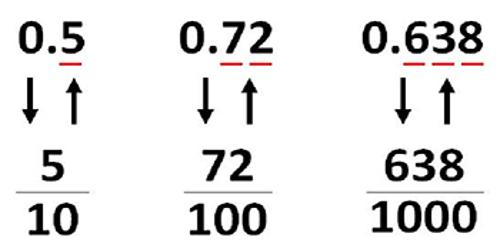Relating Decimals to Fractions

Decimals are a type of fractional number. The decimal 0.5 represents the fraction 5/10. The decimal 0.25 represents the fraction 25/100. Decimal fractions always have a denominator based on a power of 10.

We know that 5/10 is equivalent to 1/2 since 1/2 times 5/5 is 5/10. Therefore, the decimal 0.5 is equivalent to 1/2 or 2/4, etc.Some common Equivalent Decimals and Fractions:

• 1 and 1/10
• 2 and 1/5
• 5 and ½
• 25 and ¼
• 50 and ½
• 75 and ¾
• 0 and 1/1 or 2/2 or 1

Changing to a Decimal from a Fraction

Now let’s see how we change fractions into decimals. Let’s try changing 1/8 into a decimal. Notice that the slash is the same slash that we use to mean division in algebra. Remember that part and you will know how to turn a fraction into a decimal.

Yes, you guessed it! We will divide to find our decimal. Dividing 1 by 8, we get 0.125, and there we have our decimal.

To convert a Decimal to a Fraction follow these steps:

Step 1: Write down the decimal divided by 1, like this: decimal/1

Step 2: Multiply both top and bottom by 10 for every number after the decimal point. (For example, if there are two numbers after the decimal point, then use 100, if there are three then use 1000, etc.)

Step 3: Simplify (or reduce) the fraction.

Example:

How can we reduce a fraction in which the numerator or denominator is a decimal?

1.5/2

Solution: Make both terms into whole numbers by multiplying them by the same power of 10.

That is called clearing of decimals.

Clear of decimals and reduce: 1.5/2

To reduce a fraction, both the numerator and denominator must be whole numbers.  To make 1.5 into the whole number 15, multiply it by 10.  (Lesson 4, Question 2.)  Multiply 2 by 10 also:

1.5/2 = 15/20 = ¾.

15 and 20 have a common divisor 5. Now, after reducing 1.5/2, we see that, proportionally, 1.5 is to 2  as  3 is to 4.

Therefore, if we ask, “What ratio has 1.5 to 2?”, we can answer 1.5 is three fourths of 2.

Information Source: